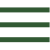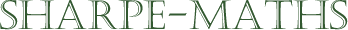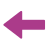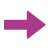Skip to main contentPrevious topic:Current topic:
Introduction to expressionsNext topic:
Collecting like terms

## Algebraic expressions

The main audience for this pack of algebraic manipulation exercises is GCSE/IGCSE revision, though I use some of the exercises with younger students; often in a different form: Tarsia jigsaws are a personal favourite and pairs/small group work with mini-whiteboards.

These materials are organised in (roughly) increasing levels of difficulty. Though the order can be changed if your scheme of work, for example, covers multiplying binomials before simple factorisation.

However, the order in which the techniques appear should not be changed too much as many techniques depend on prior ones. The exercises are likewise designed to be done more or less in the order presented, as, in general they become more difficult as they progress. If you are doing revision work you may want to concentrate on the mixed exercises which appear at various points, though for introductory work, the examples are often grouped so that patterns can be noticed by the students.

An example of this might be where factorising $x^2 + x - 6$ is followed by $x^2 - x - 6$. Throughout the booklet, $x$ has been substituted for other letters, so that students don’t say things like “I can do it with $x$ but not with $a$.” and other similar gripes. The letters have been made the same if a pattern point is being made, as above. The answers are given after each exercise (or each pair in some cases) so that students can quickly check their answers after each attempt. This is to avoid the unwelcome outcome where a student will practise the same error over and over again, and become extremely adept at making that error. (Unlearning is much harder than learning!) There are as many different ways of laying out mathematical calculations as there are teachers, so I have intentionally not suggested methods, though I may add these later in the teacher notes and lesson plans, so that you can encourage your students to do it “the right way”. It is important to note that these exercises are but one resource and it is probable that you will want to use other resources in addition, or turn some of the examples into Tarsia jigsaws. The idea behind these exercises is to give a lot of examples to use, as most text book don’t provide enough and many online exercises are created randomly using some algorithm or other and so do not point up the patterns. How you use these exercises is entirely up to you. In addition, the mixed exercises make very good consolidation or assessment materials. Most of the exercises are fairly self-explanatory, but I have added a few notes below on some of them.